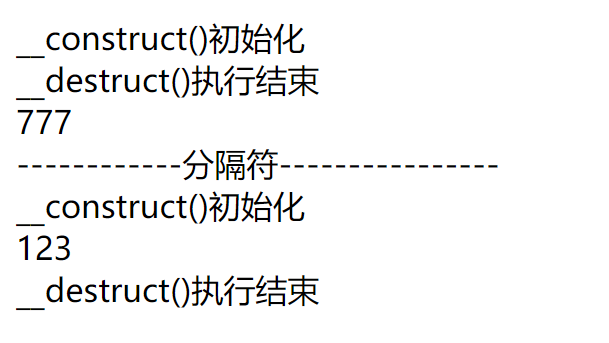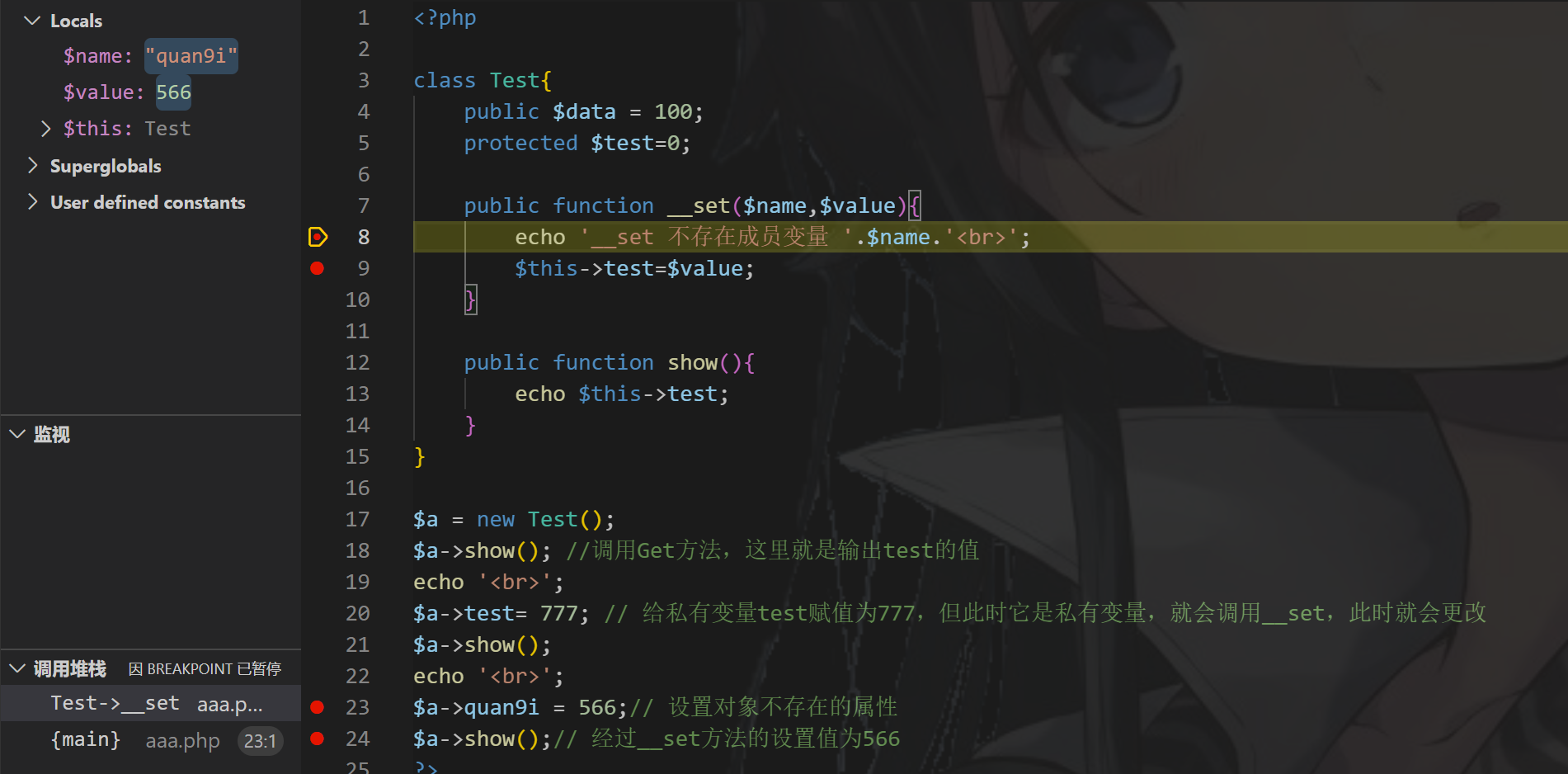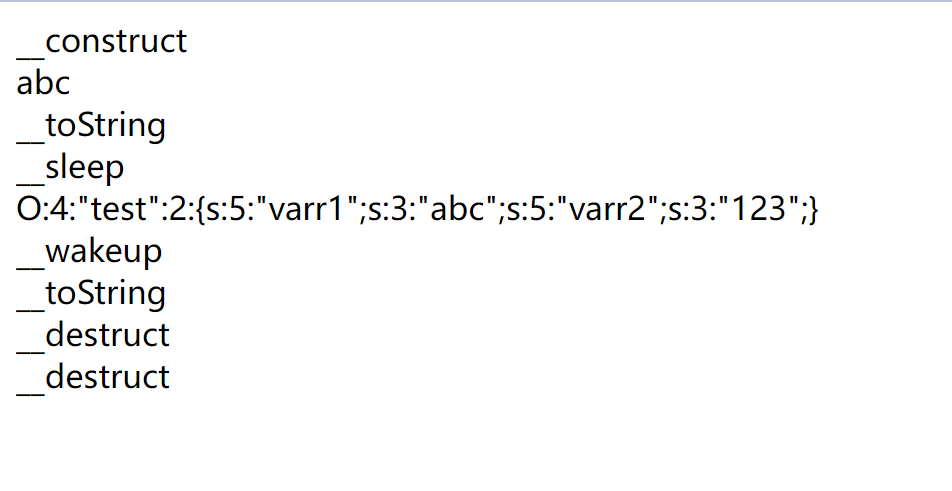# 类和对象

## 面向对象（部分）

### 组成

#### 类

``````<?php
class phpClass { //phpClass是这个类的名字，类在定义时需要加上class
var \$var1; //成员变量，名字是var1
var \$var2 = "constant string"; //成员变量

function myfunc (\$arg1, \$arg2) { //定义成员函数
[..]//函数中的内容
}
[..]
}
?>

#### 对象

``````\$mercedes = new Car ();// Car()在这里是个类名，new +类名可以实例化一个对象，这里的\$mercedes就是我们的对象名
\$bmw = new Car ();
\$audi = new Car ();``````

### 完整过程

#### 类的定义

``````<?php
class Site {
/* 成员变量 */
var \$url;
var \$title;

/* 成员函数 */
function setUrl(\$par){ //设置url方法
\$this->url = \$par;
}

function getUrl(){ //获取url方法
echo \$this->url . PHP_EOL;
}

function setTitle(\$par){ //设置标题方法
\$this->title = \$par;
}

function getTitle(){ //获取标题方法
echo \$this->title . PHP_EOL;
}
}
?>``````

#### 创建对象

``\$php = new Site; //创建了对象php``

#### 调用成员方法

``````\$php->setUrl("www.php.cn");//调用设置url方法并赋值
\$php->setTitle('PHP中文网');//调用设置title方法并赋值``````

``````\$php->getUrl();
\$php->getTitle();``````

``````<?php
class Site {
/* 成员变量 */
var \$url;
var \$title;

/* 成员函数 */
function setUrl(\$par){ //设置url方法
\$this->url = \$par;
}

function getUrl(){ //获取url方法
echo \$this->url . PHP_EOL;
}

function setTitle(\$par){ //设置标题方法
\$this->title = \$par;
}

function getTitle(){ //获取标题方法
echo \$this->title . PHP_EOL;
}
}
\$php = new Site; //创建了对象php
\$php->setUrl("www.php.cn");//调用设置url方法并赋值
\$php->setTitle('PHP中文网');//调用设置title方法并赋值
\$php->getUrl();
\$php->getTitle();
?>``````# 魔术方法

``````__construct()   当一个对象创建时被调用，
__destruct()   当一个对象销毁时被调用，
__toString()   当一个对象被当作一个字符串被调用。
__wakeup()   使用unserialize时触发
__sleep()    使用serialize时触发
__destruct()    对象被销毁时触发
__call()    在对象上下文中调用不可访问的方法时触发
__callStatic()    在静态上下文中调用不可访问的方法时触发
__get()    用于从不可访问的属性读取数据
__set()    用于将数据写入不可访问的属性
__isset()    在不可访问的属性上调用isset()或empty()触发
__unset()     在不可访问的属性上使用unset()时触发
__toString()    把类当作字符串使用时触发,返回值需要为字符串
__invoke()   当脚本尝试将对象调用为函数时触发``````

## __construct()与__destruct()

``````__construct ： 在创建对象时候初始化对象，一般用于对变量赋初值。
__destruct ： 和构造函数相反，当对象所在函数调用完毕后执行。``````
``````<?php
class bai{
public \$name;
public \$age;
public function __construct()
{
echo "__construct()初始化<br>";
\$this->name;
\$this->age;
}
public function __destruct()
{
echo "__destruct()执行结束";
}
}
\$a=new bai(); //创建一个对象命名为a
/*
赋值
\$a->name='quan9i'; //给对象a里的name赋值为quan9i
\$a->age=19; //给对象a里的age赋值为19
echo(serialize(\$a)); //输出序列化后的\$a
*/````````````<?php
class bai{
public \$name;
public \$age;
public function __construct(\$name,\$age)
{
echo "__construct()初始化<br>";
\$this->name=\$name;//将传入的第一个参数赋值给name变量
\$this->age=\$age;
}
public function __destruct()
{
echo "__destruct()执行结束<br>";
}
}
//主动销毁
\$a=new bai('quan9i',19);
unset (\$a);//主动销毁对象，此时先触发destruct魔法函数再echo
echo"777<br>";
echo "------------分隔符----------------<br>";
//自动销毁
\$b=new bai('quan9i',19);
echo "123<br>";
//此时先echo再触发destruct函数
?>``````## __sleep()

``__sleep()    serialize 之前被调用，可以指定要序列化的对象属性。``
``````<?php
class bai{
public \$name;
public \$age;
public function __construct(\$name,\$age)
{
echo "__construct()初始化<br>";
\$this->name=\$name;
\$this->age=\$age;
}
public function __sleep()
{
echo "当使用serialize时触发此方法<br>";
return array('name','age');
}
}
\$a=new bai('quan9i',19);
echo serialize(\$a);
?>``````

## __wakeup()

``__wakeup()   反序列化恢复对象之前调用该方法``

``````<?php
class bai{
public \$name;
public \$age;
public function __construct(\$name,\$age)
{
echo "__construct()初始化<br>";
\$this->name=\$name;
\$this->age=\$age;
}
public function __wakeup()
{
echo "当使用unserialize时触发此方法<br>";
\$this->age=1000;//更改\$age的值为1000
}
}
\$a=new bai('quan9i',19);
\$b= serialize(\$a);
var_dump(unserialize(\$b));
?>``````

## __toString()

``__toString() ：在对象当做字符串的时候会被调用。``

``````<?php

class Test
{
public \$a = 'This is a string';

public function good(){
echo \$this->a.'<br />';
}

// 在对象当做字符串的时候会被调用
public function __toString()
{
return '__toString方法被调用 <br>';
}
}

\$a = new Test();
\$a->good();
echo \$a;
?>``````

## __invoke()

``__invoke() ：将对象当作函数来使用时执行此方法。``

``````<?php

class Test{
public \$data ="调用正常方法<br>";

public function __invoke()
{
echo"调用__invoke()方法";
}
}
\$a= new test();
echo \$a();
?>``````

## __get

``__get() 访问不存在的成员变量时调用的``

``````<?php

class Test {
public \$n=123;
public function __get(\$name){
echo '__get方法被调用，其中不存在变量'.\$name.'<br>';
}
}
\$a = new Test();
echo \$a->quan9i;//调用对象a中的变量quan9i，由于不存在quan9i这个变量，这时候就会调用__get魔术方法
?>``````

## set

``__set() ：设置不存在的成员变量时调用的；``
``````<?php

class xg{
public \$data = 100;
protected \$test=0;

public function __set(\$name,\$value){
echo '__set 不存在成员变量 '.\$name.'<br>';
\$this->test=\$value;
}

public function show(){
echo \$this->test;
}
}

\$a = new xg();
\$a->show(); //调用Get方法，这里就是输出test的值
echo '<br>';
\$a->test= 777; // 给私有变量test赋值为777，但此时它是私有变量，就会调用__set，此时就会更改
\$a->show();
echo '<br>';
\$a->quan9i = 566;// 设置对象不存在的属性
\$a->show();// 经过__set方法的设置值为566
?>``````## __call()

``__call ：当调用对象中不存在的方法会自动调用该方法``

``````<?php

class Test{
public \$data ="调用正常方法<br>";
public function __call(\$name,\$value){
echo "__call被调用，Test类中不存在方法".\$name.'<br>';
var_dump(\$value);
}
public function show(){
echo \$this->data;
}
}
\$a= new test();
\$a->show(); //调用正常方法
echo"--------------------分隔符----------------<br>";
\$a->quan9i('quan9i',123); //调用一个不存在的方法，此时就调用了__call魔术方法

?>``````

## isset()

``__isset() : 检测对象的某个属性是否存在时执行此函数。``

``````<?php

class test{
public \$name;
private \$age;

public function __construct(\$name, \$age){
\$this->name = \$name;
\$this->age = \$age;
}

// __isset()：当对不可访问属性调用 isset() 或 empty() 时，__isset() 会被调用。
public function __isset(\$content){
echo "当使用isset()函数，自动调用<br>";
return isset(\$this->\$content);
}
}

\$a = new test("quan9i", 19);
// public 成员
echo (\$a->name),"<br>";
// private 成员
echo isset(\$a->name);
echo "-----------分隔符--------------<br>";
echo isset(\$a->age);
?>``````## __unset()

``__unset() ：在不可访问的属性上使用 unset () 时触发``

``````<?php

class test{
public \$name;
private \$age;

public function __construct(\$name, \$age){
\$this->name = \$name;
\$this->age = \$age;
}

public function __unset(\$content){
echo "当使用unset()函数，自动调用<br>";
echo "quan9i will be the best";
}
}

\$a = new test("quan9i", 19);
// public 成员
unset(\$a->name);
echo "<br>";
// private 成员
echo "-----------分隔符--------------<br>";
unset(\$a->age);
echo "<br>";
?>``````

# 常用魔术函数汇总例子

``````<?php
class test{
public \$varr1="abc";
public \$varr2="123";
public function echoP(){
echo \$this->varr1."<br>";
}
public function __construct(){
echo "__construct<br>";
}
public function __destruct(){
echo "__destruct<br>";
}
public function __toString(){
return "__toString<br>";
}
public function __sleep(){
echo "__sleep<br>";
return array('varr1','varr2');
}
public function __wakeup(){
echo "__wakeup<br>";
}
}

\$obj = new test();  //实例化对象，调用__construct()方法，输出__construct
\$obj->echoP();   //调用echoP()方法，输出"abc"
echo \$obj;    //obj对象被当做字符串输出，调用__toString()方法，输出__toString
\$s =serialize(\$obj);  //obj对象被序列化，调用__sleep()方法，输出__sleep
echo \$s.'<br>';//sleep返回的信息此时被输出
echo unserialize(\$s);  //\$s首先会被反序列化，会调用__wake()方法，被反序列化出来的对象又被当做字符串，就会调用_toString()方法。后面这个是一个对象，因此会执行一次__destruct方法
// 结束又会调用__destruct()方法，输出__destruct
?>``````https://spaceman-911.gitee.io/2021/06/30/PHP反序列化/

## 发送评论编辑评论

|´・ω・)ノ
ヾ(≧∇≦*)ゝ
(☆ω☆)
（╯‵□′）╯︵┴─┴
￣﹃￣
(/ω＼)
∠( ᐛ 」∠)＿
(๑•̀ㅁ•́ฅ)
→_→
୧(๑•̀⌄•́๑)૭
٩(ˊᗜˋ*)و
(ノ°ο°)ノ
(´இ皿இ｀)
⌇●﹏●⌇
(ฅ´ω`ฅ)
(╯°A°)╯︵○○○
φ(￣∇￣o)
ヾ(´･ ･｀｡)ノ"
( ง ᵒ̌皿ᵒ̌)ง⁼³₌₃
(ó﹏ò｡)
Σ(っ °Д °;)っ
( ,,´･ω･)ﾉ"(´っω･｀｡)
╮(╯▽╰)╭
o(*////▽////*)q
＞﹏＜
( ๑´•ω•) "(ㆆᴗㆆ)

Emoji

...